Home | Study Links | Poems |

## A Cubical Universe, a New Prime Numbers HypothesisWhile searching for prime number patterns, I imagined a line length consisting of an infinite number of evenly
spaced prime numbers (1 to infinity + 1), where infinity = 0, which implies a reversal of numbers.
With other words, the beginning is the end, and the end is the beginning.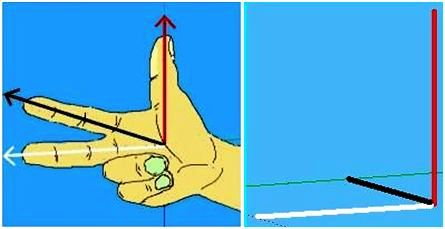Naturally the ends of this line must relate to a mirror image of itself, which brought into play the idea of Fleming's right-hand rule, which shows the relationship of electromagnetic currents and motion. The thumb represents motion, the forefinger magnetic field, and the middle finger electric current at a right angle to the forefinger, so that all are set at right angles to each other. This is one out of eight identical triune corner pieces.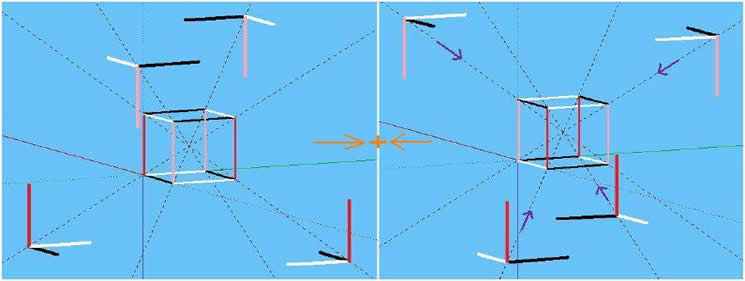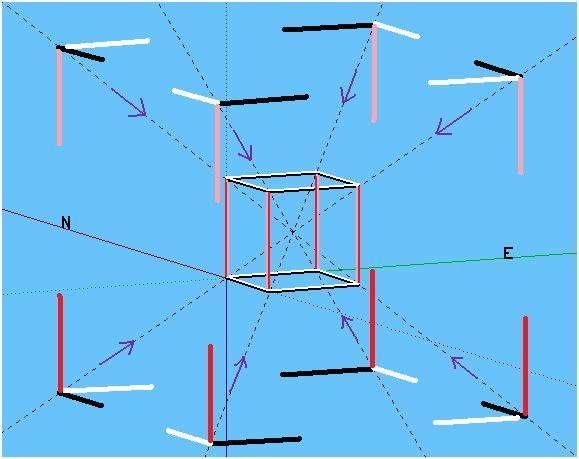Together there are 12 lines making up this cube frame with 12 lines mirroring it.I propose, the cosmos is comprised of a harmonious grid matrix, interwoven by electromagnetic spectrum
frequency waves comprised of non-prime numbers like finely woven threads of fabric, where prime numbers in this grid matrix are like pockets encapsulated by the non-prime numbered frequencies, and represent things visible.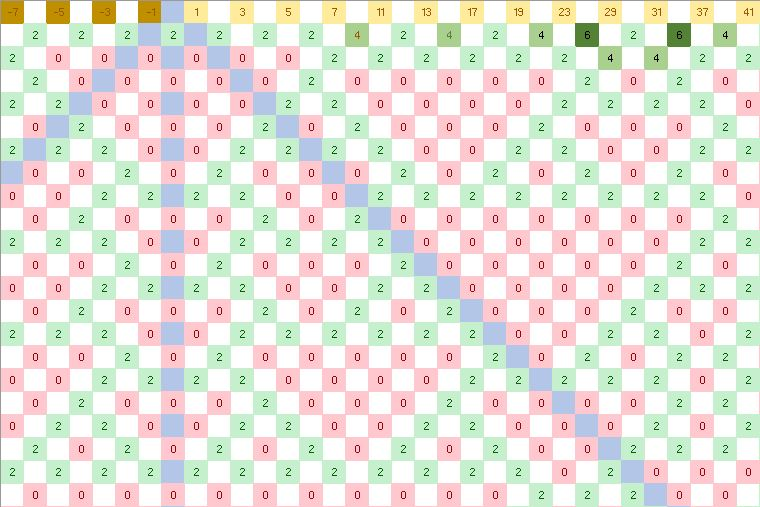The prime numbers reflect a unique and rock-solid pattern.
Logically, the pattern (0’s and 2’s) carrying those prime numbers cannot be out by one iota.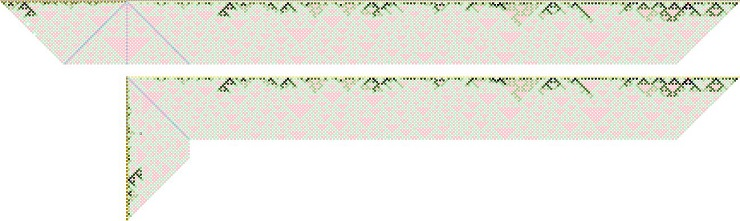First 150 or so prime numbers, and showing the fold.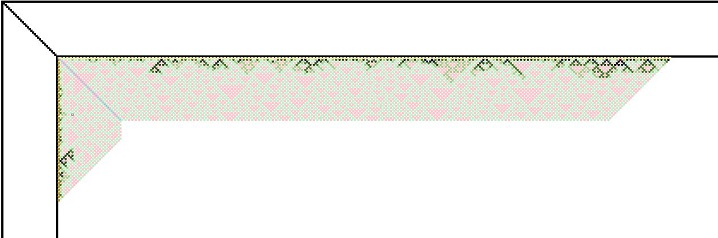The cube frame edges have condensed patterns like waves on the surface of an ocean.
Imagine this as concentrated energy, visible matter, or various sized objects floating on water.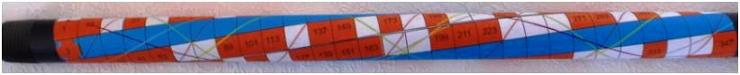The frame edges comprised of prime number frequencies as explained in Primes and Patterns,
are accurately depicted in this image as tubular, circular, and spiralling, instead of flat and straight.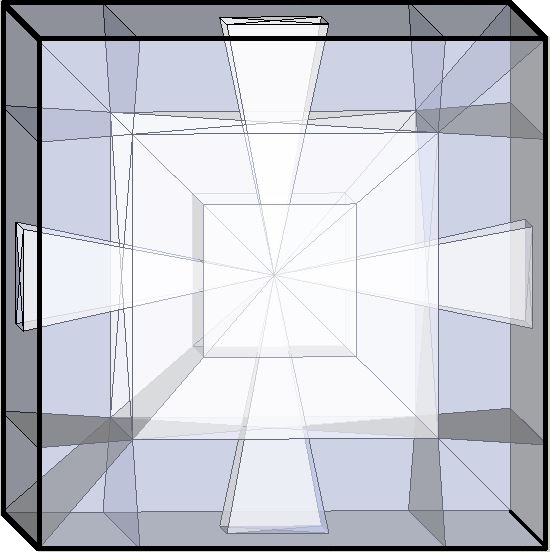The cube represents an infinite universe. For easier viewing I omitted the front and back cones.
For more ideas on this multi-dimensional drawing see the Sun Square page.Picture this cube idea as spherical (atom or universe) where the information originates from the center.

Perhaps, the universe consists of a small percentage visible substance, with the rest comprised of an invisible grid matrix and frequency waves (dark matter and dark energy?), and operates with computer like precision,
using digital processes; bits like the 1’s and 0’s of computers, portrayed here as 0’s and 2’s.

Logically, an expanding cosmos generates pre-existing  ever-growing numbers, frequencies, patterns, and primes.

An afterthought idea, but maybe the cubical/sphere configuration and the relationship of electromagnetic currents and motion as described above, is a pattern for a levitating craft where the electromagnetic force is able to manipulate the gravitational force in all directions.
The only force you need to counter is the electromagnetic force Nikola Tesla